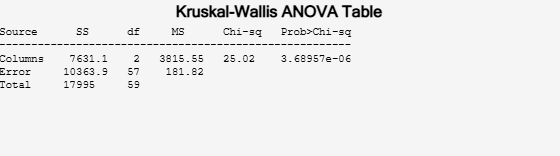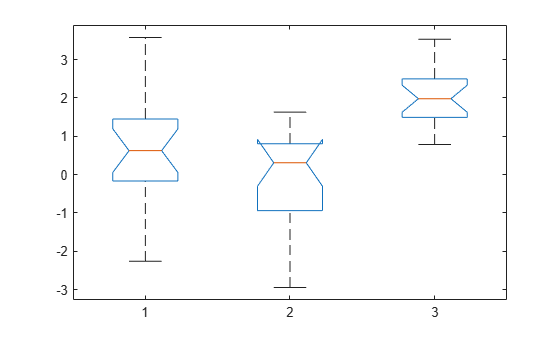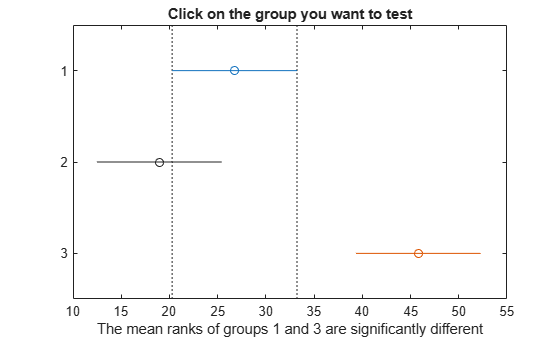# kruskalwallis

Kruskal-Wallis test

## Syntax

``p = kruskalwallis(x)``
``p = kruskalwallis(x,group)``
``p = kruskalwallis(x,group,displayopt)``
``````[p,tbl,stats] = kruskalwallis(___)``````

## Description

example

````p = kruskalwallis(x)` returns the p-value for the null hypothesis that the data in each column of the matrix `x` comes from the same distribution, using a Kruskal-Wallis test. The alternative hypothesis is that not all samples come from the same distribution. `kruskalwallis` also returns an ANOVA table and a box plot.```

example

````p = kruskalwallis(x,group)` returns the p-value for a test of the null hypothesis that the data in each categorical group, as specified by the grouping variable `group` comes from the same distribution. The alternative hypothesis is that not all groups come from the same distribution.```

example

````p = kruskalwallis(x,group,displayopt)` returns the p-value of the test and lets you display or suppress the ANOVA table and box plot.```

example

``````[p,tbl,stats] = kruskalwallis(___)``` also returns the ANOVA table as the cell array `tbl` and the structure `stats` containing information about the test statistics.```

## Examples

collapse all

Create two different normal probability distribution objects. The first distribution has `mu = 0` and `sigma = 1`, and the second distribution has `mu = 2` and `sigma = 1`.

```pd1 = makedist('Normal'); pd2 = makedist('Normal','mu',2,'sigma',1);```

Create a matrix of sample data by generating random numbers from these two distributions.

```rng('default'); % for reproducibility x = [random(pd1,20,2),random(pd2,20,1)];```

The first two columns of `x` contain data generated from the first distribution, while the third column contains data generated from the second distribution.

Test the null hypothesis that the sample data from each column in `x` comes from the same distribution.

`p = kruskalwallis(x)````p = 3.6896e-06 ```

The returned value of `p` indicates that `kruskalwallis` rejects the null hypothesis that all three data samples come from the same distribution at a 1% significance level. The ANOVA table provides additional test results, and the box plot visually presents the summary statistics for each column in `x`.

Create two different normal probability distribution objects. The first distribution has `mu = 0` and `sigma = 1`. The second distribution has `mu = 2` and `sigma = 1`.

```pd1 = makedist('Normal'); pd2 = makedist('Normal','mu',2,'sigma',1);```

Create a matrix of sample data by generating random numbers from these two distributions.

```rng('default'); % for reproducibility x = [random(pd1,20,2),random(pd2,20,1)];```

The first two columns of `x` contain data generated from the first distribution, while the third column contains data generated from the second distribution.

Test the null hypothesis that the sample data from each column in `x` comes from the same distribution. Suppress the output displays, and generate the structure `stats` to use in further testing.

`[p,tbl,stats] = kruskalwallis(x,[],'off')`
```p = 3.6896e-06 ```
```tbl=4×6 cell array Columns 1 through 5 {'Source' } {'SS' } {'df'} {'MS' } {'Chi-sq' } {'Columns'} {[7.6311e+03]} {[ 2]} {[3.8155e+03]} {[ 25.0200]} {'Error' } {[1.0364e+04]} {} {[ 181.8228]} {0x0 double} {'Total' } {[ 17995]} {} {0x0 double } {0x0 double} Column 6 {'Prob>Chi-sq'} {[ 3.6896e-06]} {0x0 double } {0x0 double } ```
```stats = struct with fields: gnames: [3x1 char] n: [20 20 20] source: 'kruskalwallis' meanranks: [26.7500 18.9500 45.8000] sumt: 0 ```

The returned value of `p` indicates that the test rejects the null hypothesis at the 1% significance level. You can use the structure `stats` to perform additional followup testing. The cell array `tbl` contains the same data as the graphical ANOVA table, including column and row labels.

Conduct a followup test to identify which data sample comes from a different distribution.

`c = multcompare(stats);`
```Note: Intervals can be used for testing but are not simultaneous confidence intervals. ```Display the multiple comparison results in a table.

```tbl = array2table(c,"VariableNames", ... ["Group A","Group B","Lower Limit","A-B","Upper Limit","P-value"])```
```tbl=3×6 table Group A Group B Lower Limit A-B Upper Limit P-value _______ _______ ___________ ______ ___________ __________ 1 2 -5.1435 7.8 20.744 0.33446 1 3 -31.994 -19.05 -6.1065 0.0016282 2 3 -39.794 -26.85 -13.906 3.4768e-06 ```

The results indicate that there is a significant difference between groups 1 and 3, so the test rejects the null hypothesis that the data in these two groups comes from the same distribution. The same is true for groups 2 and 3. However, there is not a significant difference between groups 1 and 2, so the test does not reject the null hypothesis that these two groups come from the same distribution. Therefore, these results suggest that the data in groups 1 and 2 come from the same distribution, and the data in group 3 comes from a different distribution.

Create a vector, `strength`, containing measurements of the strength of metal beams. Create a second vector, `alloy`, indicating the type of metal alloy from which the corresponding beam is made.

```strength = [82 86 79 83 84 85 86 87 74 82 ... 78 75 76 77 79 79 77 78 82 79]; alloy = {'st','st','st','st','st','st','st','st',... 'al1','al1','al1','al1','al1','al1',... 'al2','al2','al2','al2','al2','al2'};```

Test the null hypothesis that the beam strength measurements have the same distribution across all three alloys.

`p = kruskalwallis(strength,alloy,'off')`
```p = 0.0018 ```

The returned value of `p` indicates that the test rejects the null hypothesis at the 1% significance level.

## Input Arguments

collapse all

Sample data for the hypothesis test, specified as a vector or an m-by-n matrix. If `x` is an m-by-n matrix, each of the n columns represents an independent sample containing m mutually independent observations.

Data Types: `single` | `double`

Grouping variable, specified as a numeric or logical vector, a character or string array, or a cell array of character vectors.

• If `x` is a vector, then each element in `group` identifies the group to which the corresponding element in `x` belongs, and `group` must be a vector of the same length as `x`. If a row of `group` contains an empty value, that row and the corresponding observation in `x` are disregarded. `NaN` values in either `x` or `group` are similarly ignored.

• If `x` is a matrix, then each column in `x` represents a different group, and you can use `group` to specify labels for these columns. The number of elements in `group` and the number of columns in `x` must be equal.

The labels contained in `group` also annotate the box plot.

Example: `{'red','blue','green','blue','red','blue','green','green','red'}`

Data Types: `single` | `double` | `logical` | `char` | `string` | `cell`

Display option, specified as `'on'` or `'off'`. If `displayopt` is `'on'`, `kruskalwallis` displays the following figures:

• An ANOVA table containing the sums of squares, degrees of freedom, and other quantities calculated based on the ranks of the data in `x`.

• A box plot of the data in each column of the data matrix `x`. The box plots are based on the actual data values, rather than on the ranks.

If `displayopt` is `'off'`, `kruskalwallis` does not display these figures.

If you specify a value for `displayopt`, you must also specify a value for `group`. If you do not have a grouping variable, specify `group` as `[]`.

Example: `'off'`

## Output Arguments

collapse all

p-value of the test, returned as a scalar value in the range [0,1]. `p` is the probability of observing a test statistic as extreme as, or more extreme than, the observed value under the null hypothesis. Small values of `p` cast doubt on the validity of the null hypothesis.

ANOVA table of test results, returned as a cell array. `tbl` includes the sums of squares, degrees of freedom, and other quantities calculated based on the ranks of the data in `x`, as well as column and row labels.

Test data, returned as a structure. You can perform followup multiple comparison tests on pairs of sample medians by using `multcompare`, with `stats` as the input value.

collapse all

### Kruskal-Wallis Test

The Kruskal-Wallis test is a nonparametric version of classical one-way ANOVA, and an extension of the Wilcoxon rank sum test to more than two groups. The Kruskal-Wallis test is valid for data that has two or more groups. It compares the medians of the groups of data in `x` to determine if the samples come from the same population (or, equivalently, from different populations with the same distribution).

The Kruskal-Wallis test uses ranks of the data, rather than numeric values, to compute the test statistics. It finds ranks by ordering the data from smallest to largest across all groups, and taking the numeric index of this ordering. The rank for a tied observation is equal to the average rank of all observations tied with it. The F-statistic used in classical one-way ANOVA is replaced by a chi-square statistic, and the p-value measures the significance of the chi-square statistic.

The Kruskal-Wallis test assumes that all samples come from populations having the same continuous distribution, apart from possibly different locations due to group effects, and that all observations are mutually independent. By contrast, classical one-way ANOVA replaces the first assumption with the stronger assumption that the populations have normal distributions.

## Version History

Introduced before R2006a